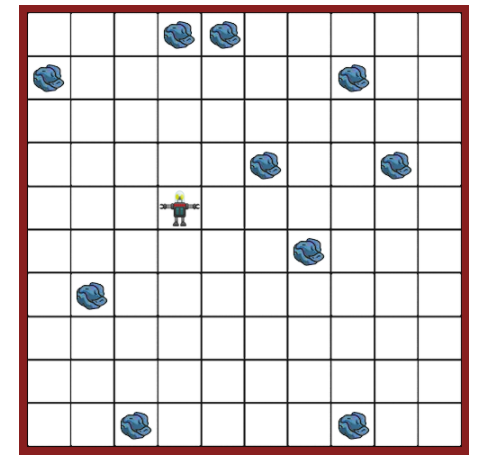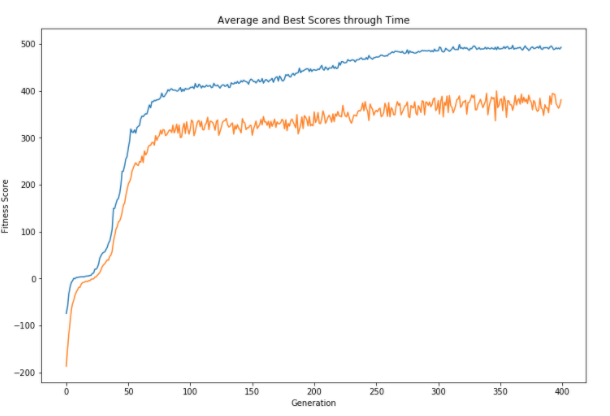## python遗传算法的优化过程

282次阅读l 生成问题初始随机解的“种群”

l 个体的“拟合度”是根据它解决问题的程度来评估的

l 最合适的解决方案进行“繁殖”并将“遗传”物质传递给下一代的后代

l 重复第2步和第3步，直到我们得到一组优化的解决方案、

```# 初始种群
pop = [Robot() for x in range(pop_size)]
results = []

# 执行进化
for i in tqdm(range(num_gen)):
scores = np.zeros(pop_size)

# 遍历所有机器人
for idx, rob in enumerate(pop):
# 运行垃圾收集模拟并计算拟合度
score = rob.simulate(iter_per_sim, moves_per_iter)
scores[idx] = score

results.append([scores.mean(),scores.max()]) # 保存每一代的平均值和最大值

best_robot = pop[scores.argmax()] # 保存最好的机器人

# 限制那些能够交配的机器人的数量
inds = np.argpartition(scores, -num_breeders)[-num_breeders:] # 基于拟合度得到顶级机器人的索引
subpop = []
for idx in inds:
subpop.append(pop[idx])
scores = scores[inds]

# 平方并标准化
norm_scores = (scores - scores.min()) ** 2
norm_scores = norm_scores / norm_scores.sum()

# 创造下一代机器人
new_pop = []
for child in range(pop_size):
# 选择拟合度优秀的父母
p1, p2 = np.random.choice(subpop, p=norm_scores, size=2, replace=False)
new_pop.append(Robot(p1.dna, p2.dna))

pop = new_pop```